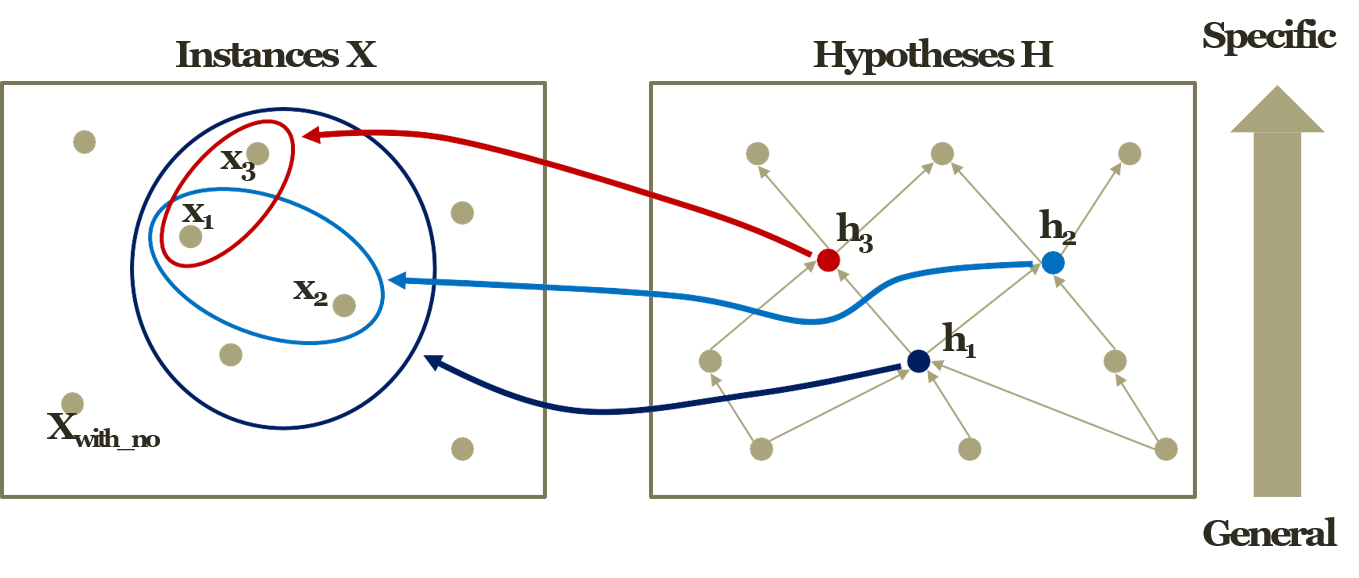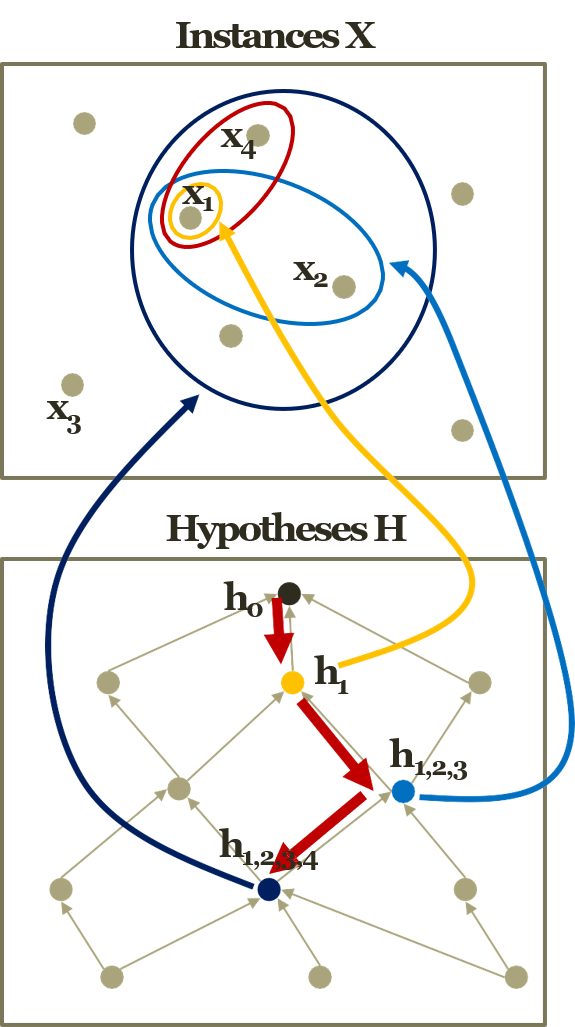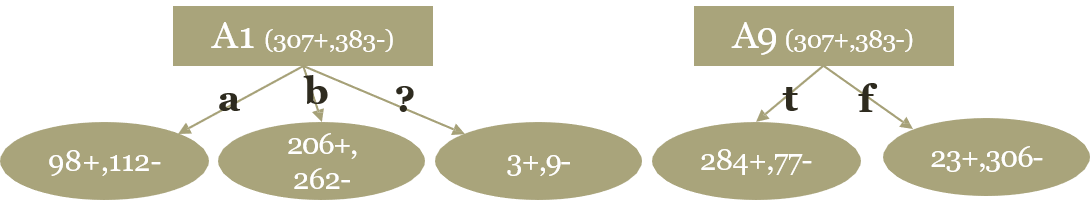# Decision Tree (Reference ML KAIST)

2018, Aug 28

Before dividing into Decision Tree, what is machine learning?

• A computer program is said that
• learn from experience E
• With respect to some class of tasks T
• And performance measure P, if its performance at tasks in T, as measured by P, improves with experience E

### A Perfect World for Rule Based Learning

Imagine a perfect world with

• No observation errors, No inconsistent observations.
• (Training data is error-free, noise-free)
• No stochastic elements in the system we observe.
• (Target function is deterministic)
• Full information in the observations to regenerate the system
• (Target function is contained in hypotheses set)

Observation of the people

• Sky, Temp, Humid, Wind, Water, Forecast → EnjoySport
Sky Temp Humid Wind Water Forecast EnjoySport
Sunny Warm Normal Strong Warm Same Yes
Sunny Warm High Strong Warm Same Yes
Rainy Cold High Strong Warm Change No
Sunny Warm High Strong Cool Change Yes

### Function Approximation

Machine learning is relative to applying function approximation well.

• Machine Learning ?
• The effort of producing a better approximate function
• In the perfect world of EnjoySport
• Instance X
• Features : <Sunny, Warm, Normal, Strong, Warm, Same>
• Label : Yes
• Training dataset D
• A collection of observations on the instance (Collection of Xs)
• Hypothesis H
• Potentially possible function to turn X into Y
• h_i : <Sunny, Warm, ?, ?, ?, Same> → Yes
• Target function C
• Unknown target function between the features and the label
• Our objective is expressing hypothesis H to target function C.

### Graphical Representation of Function Approximation• x1 : <Sunny, Warm, Normal, Strong, Warm, Same>, h1 : <Sunny, ?, ?, ?, Warm, ?>
• x2 : <Sunny, Warm, Normal, Light, Warm, Same>, h2 : <Sunny, ?, ?, ?, Warm, Same>
• x3 : <Sunny, Warm, Normal, Strong, Warm, Change>, h3 : <Sunny, ?, ?, Strong, Warm, ?>

• What would be the better function approximation?
• Generalization Vs. Specialization
• Instance x1 & Hypothesis h1 is Generalization
• Instance x3 & Hypothesis h3 is Specialization

### Find-S Algorithm• Find-S Algorithm
• Initialize h to the most specific in H
• For instance x in D
• if x is positive
• For feature f in O
• if $f_{i}$ in h == $f_{i}$ in x
• Do nothing
• else
• ($f_{i}$ in h) ← ($f_{i}$ in h) $\cup$ ($f_{i}$ in x)
• Return h

For example,

• Instances
• $x_{1}$ : <Sunny, Warm, Normal, Strong, Warm, Same>
• $x_{2}$ : <Sunny, Warm, Normal, Light, Warm, Same>
• $x_{4}$ : <Sunny, Warm, Normal, Strong, Warm, Change>
• Hypothesis
• $h_{0}$ = $<\varnothing, \varnothing, \varnothing, \varnothing, \varnothing \varnothing>$
• $h_{1}$ = <Sunny, Warm, Normal, Strong, Warm, Same>
• $h_{1,2}$ = <Sunny, Warm, Normal, Don’t care, Warm, Same>
• $h_{1,2,4}$ = <Sunny, Warm, Normal, Don’t care, Warm, Don’t care>
• Any possible problems?
• Many possible hs, and can’t determine the coverage.

### we live with noises

• We need a better learning method
• We need to have more robust methods given the noises
• We need to have more concise presentations of the hypotheses
• One alternative is a decision tree.Good decision tree split the data as good ratio. Above example, A1 or A9 are features. In the case of A9, we have two options, True/False. If we choose True(left) then, we get correct answer 284/307. otherwise, 306/383, which is not good but not bad result.

In the decision tree, we should consider how to split the data in order to get good results.

### Entropy

• Better attribute to check?
• Reducing the most uncertainty
• Then, how to measure the uncertainty of a feature variable
• Entropy of a random variable
• Features are random variables
• Higher entropy means more uncertainty
• $H(x) = -\sum_{x}P(X=x)H(X=x)$
• Conditional Entropy
• We are interested in the entropy of the class given a feature variable
• Need to introduce a given condition in the entropy
• $H(Y|X) = \sum_{x}P(X = x)H(Y | X = x) = \sum_{x}P(X = x){-\sum_{Y}P(Y = y | X = x)log_{b}P(Y = y | X = x)}$